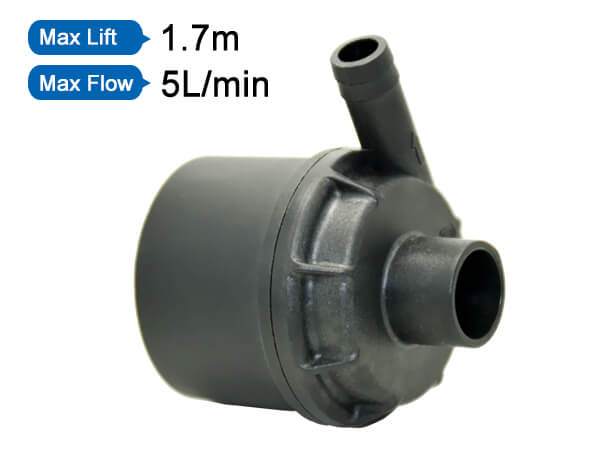### Shenpeng Electronics

Water Pump to provide solutions

+86-769-81868638

Published:2021-10-15What is traffic?

The volume of the liquid discharged by the pump per unit time is called the flow rate, and the flow rate is expressed by Q. The unit of measurement: cubic meter/hour (m3/h), liter/second (l/s), L/s=3.6 m3/h=0.06 m3/ min=60L/minG=Qρ G is the weight ρ is the specific gravity of the liquid

Example: The flow rate of a certain pump is 50 m3/h. What is the weight per hour when pumping water? The specific gravity ρ of water is 1000 kg/m3. Solution: G=Qρ=50×1000(m3/h·kg/ m3)=50000kg / h=50t/h

The energy obtained by the unit weight of the liquid through the pump is called the head. The head of the pump, including the suction stroke, is approximately equal to the pressure difference between the outlet and inlet of the pump. The head is represented by H, and the unit is meter (m). The pressure of the pump is expressed by P, the unit is Mpa (megapascals), H=P/ρ. If P is 1kg/cm2, then H=(lkg/ cm2)/(1000kg/ m3) H=(1kg/ cm2)/ (1000kg/m3)=(10000kg/m2)/1000kg/m3=10m1Mpa=10kg/cm2, H=(P2-P1)/ρ (P2=outlet pressure P1=inlet pressure)

What is the characteristic curve of the pump?

The curve that shows the relationship between the main performance parameters is usually called the performance curve or characteristic curve of the centrifugal pump. In essence, the performance curve of the centrifugal pump is the external manifestation of the law of liquid movement in the pump, which is obtained through actual measurement. The characteristic curves include: flow-head curve (QH), flow-efficiency curve (Q-η), flow-power curve (QN), flow-cavitation margin curve (Q-(NPSH)r), the function of the performance curve is For any flow point of the pump, a set of relative head, power, efficiency and NPSH values can be found on the curve. This set of parameters is called working state, referred to as working condition or working point, centrifugal pump The operating condition at the highest efficiency point is called the optimal operating point, and the optimal operating point is generally the design operating point. Generally, the rated parameters of centrifugal pumps are the design operating point and the optimal operating point coincide or very close to each other. It is very important to understand the performance parameters of the pump to run in the practical efficiency range, which saves energy and can ensure the normal operation of the pump. To

What is the efficiency of the pump? formula? Refers to the ratio of the effective power of the pump to the shaft power.

η=Pe/P The power of a pump usually refers to the input power, that is, the power transmitted from the prime mover to the pump shaft, so it is also called shaft power, which is represented by P. The effective power is: the product of the pump's head, mass flow and gravitational acceleration. Pe=ρg QH (W) or Pe=γQH/1000 (KW) ρ: the density of the liquid delivered by the pump (kg/m3) γ: the weight of the liquid delivered by the pump γ=ρg (N/m3)

g: gravity acceleration (m/s) mass flow Qm=ρQ (t/h or kg/s)

News
service telephone:0769-81868638